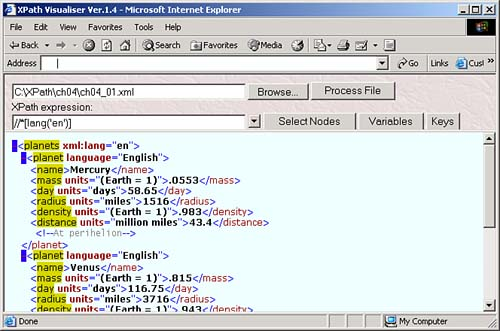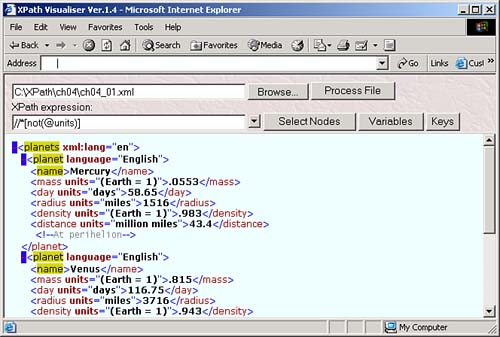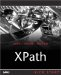The XPath Boolean Operators and Functions

You can use XPath logical operators to produce Boolean true/false results. These are the logical operators:

• != means "is not equal to."

• < means "is less-than " (use &lt; in XML documents).

• <= means "is less-than or equal to" (use &lt;= in XML documents).

• = means "is equal to" (C, C++, Java, and JavaScript programmers take notethis operator is one = sign, not two).

• > means "is greater-than."

• >= means "is greater-than or equal to."

And as we saw in Chapter 3, you can also use the and and or operators to connect Boolean clauses in predicates.

USING < IN DOCUMENTS

You shouldn't use < directly in XML documents, so you should use the entity reference &lt; instead. On the other hand, some software isn't going to understand &lt; , so you'll have to use < instead.

Here's an example using the logical operator > . This XPath location path selects all <planet> elements after the third one:

//planet[position() > 3]

XPath also supports a set of Boolean functions:

• boolean() converts its argument to a Boolean value.

• false() returns a value of false .

• lang() tests whether the language tag set with the xml:lang attribute is the same as the language passed to this function.

• not() reverses the true/false value of its argument.

• true() returns a value of true .

We'll take a look at these in more detail, starting with the boolean function.

The boolean Function

You use the boolean function to convert the argument you pass to it into a Boolean value. Here's what happens when you pass arguments of various XPath types to this function:

• boolean The same value is returned.

• number If the number is zero, the result is false . Otherwise the result is true .

• string If the string is empty, the result is false ; otherwise, the result is true .

• node-set Empty node-set returns false, otherwise returns true .

The false Function

You can use the false function to return a value of false . That's really all there is to this function.

When do you use this function? XPath does not define any Boolean constants, so if you need to assign a value of false to an XSLT variable, you can use the false function.

The lang Function

The lang function tests whether the language of the context node (as defined by the xml:lang attribute) is the same as the language you pass to it. You pass this function a string that corresponds to a language tag in the XML specification, such as "en" for English, "de" for German, or "fr" for French.

Here's an example where we're checking to make sure that the source document was written in English. We'll start by copying over our planetary data XML document, ch03_01.xml , to ch04_01.xml in the downloadable code for this book so we can use it in this chapter. Then we set the xml:lang attribute in ch04_01.xml to English like this:

<?xml version="1.0" encoding="UTF-8"?>  <planets  xml:lang="en">  <planet>         <name>Mercury</name>         <mass units="(Earth = 1)">.0553</mass>         <day units="days">54.65</day>         <radius units="miles">1516</radius>         <density units="(Earth = 1)">.983</density>         <distance units="million miles">43.4</distance><!--At perihelion-->     </planet>         .         .         .

You can see how we test the lang function with the XPath Visualiser and the location path //*[lang("en")] , which selects elements that have xml:lang set to "en". You can see the results in Figure 4.1because we've set xml:lang to "en" in the document element, <planets> , all enclosed elements also have xml:lang set to "en".

Figure 4.1. Using lang('en') .Here's an example using the lang function in XSLT. Say that in this case you want to make sure that a source document's language is English before applying templates. You can use the lang function and the <xsl:if> element like this in XLST:

<?xml version="1.0" encoding="UTF-8"?> <xsl:stylesheet version="1.1" xmlns:xsl="http://www.w3.org/1999/XSL/Transform">     <xsl:template match="/planets">  <xsl:if test="lang('en')">  <HTML>         .         .         .         </HTML>  </xsl:if>  </xsl:template>         .         .         . </xsl:stylesheet>

The not Function

You use the not function to reverse the Boolean value of the argument you pass to it. If you pass it an argument whose logical value is true , this function will return false , and if you pass it a false argument, it returns true .

You can see an example in the XPath Visualiser in Figure 4.2, where we're selecting elements that do not have a units attribute, using the location path //*[not(@units)] .

Figure 4.2. Using the not function while selecting elements.Here's an XSLT example. In this case, we'll create two templates, one for <planet> elements that have both color and rings attributes, and another template for all other <planet> elements. That looks like this:

<?xml version="1.0" encoding="UTF-8"?> <xsl:stylesheet version="1.1" xmlns:xsl="http://www.w3.org/1999/XSL/Transform">  <xsl:template match="planet[@color and @rings]">   .   .   .   </xsl:template>   <xsl:template match="planet[not(@color) or not(@rings)]">   .   .   .   </xsl:template>  </xsl:stylesheet>

The true() Function

The true function returns a value of true . And that's all it does.

XPath does not define any Boolean constants, so if you need to assign a value of true to an XSLT variable, you can use the true function.XPath Kick Start: Navigating XML with XPath 1.0 and 2.0
ISBN: 0672324113
EAN: 2147483647
Year: 2002
Pages: 131

Similar book on Amazon